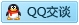# 一年级数学上册期末复习题

2015小学数学一年级上册期末考试题

5、下图中长方形有（ ）个；正方形有（ ）个；三角形有（ ）

6、

）个珠子。

7

： ） （ ： ）二、 计算：（24分）

8+7=8-6=6+10=9+4=7+5=8+5= 2+8=9+6=7-3= 9+9=4+8=10+5=

10-6=9-7=5+8= 9-4=11-10=4+5= 7-7=6+9=9+3=14-4=9+8=2+8=

1

1 8 2

20

9

5个。

○○○○○○

。 3

3个。

□ ○ □ 〓 □

□ ○ □

〓 □

8 － □ 〓 □ 8 － □ 〓 □

6

＋ □ ＝ □ 2 ＋ □ ＝ □

4、（20）（18）（17）（15）（15）（12）（6）（3） 5、（5）（2）（6）（1） 6、（3）略

7、（8：00）（1：30）（4：00）（7：30）

2

3

7+5=12 7+4=11

1．1个十是（）个一。

2．1个十和6个一组成的数是（ ）。 3．18里面有（）个十和（）个一。 4．5个一和1个十组成（）。

5．从右边起，第一位是（）位，第二位是（）位。 6．17这个数，个位上是（），十位上是（）。

7．13这个数，个位上的3表示（ ），十位上的1表示（ 8．16后面的第四个数是（ ）。

9．17和19中间的数是（ ），15后面的三个数是（ ）、（ ）、（10．和14相邻的数是（ ）和（ ） 11．20里面有（）个十。

12．2连续加2：2、（ ）、（ ）、（）、（）、（）、（）、（13．按数的顺序填空。

14．数一数。

。）。）。）

15．在○里填上“�”“�”或“�”。

15○10 18○14 12○16 10＋3○13 19○10＋10 2＋10○14 7○18－14 12＋4○17－2 16．在（ ）里填上合适的数。

（ ）＋10=164＋（ ）=14 6＋（ ）�16 1＋（ ）=18 （ ）＋6=1610＋（ ）=19 （ ）＋10=14（ ）＋6=16 （ ）－5－2=3 （ ）＋（ ）＋（ ）=17 10－（ ）－（ ）=4 二、我会算。

15－5= 19－10= 20－10= 19－9= 8＋2=12-10=5＋10= 10＋1= 8＋11= 16－10= 13－3= 18－8= 7＋10= 17－7=18－2= 10＋10= 8＋2＋1= 4＋6＋2=1＋9＋3= 3＋7＋4= 17－7－3= 10－5＋4= 15－10＋2=11－8－1= 19－9－6=

（ ）个十和 （ ）个十和 （ ）个十和 （ ）个一 （ ）个一（ ）个一

（ ）个十或（ ）个十和（ ）个十和 （ ）个一 （ ）个一（ ）个一 四、用数学。 （1）看图填空。

（2）解决问题。 ①

（只）

A．青蛙一共买了多少枝铅笔？ B．小鸭一共买了多少枝铅笔？

1 、

2、画画填填。

■■■■■■■■■＿＿＿

9+（ ）=10

）=9）=8

???＿＿＿＿＿＿＿＿ 3+（ ?????＿＿＿＿＿＿ 5+（

3、 4、 5、

6前面一个数是（），后面一个数是（）。 和8相邻的两个数是（）和（ ）。 比5大比10小的数有（ ）。

6、 ???????????有（ ）个，?有（ ）个。

??????? ?比?多（ ）个，?比?少（）个。

7

8 的右面填6

8、在?里填＜、＞或=

10?8 6?6 8?6+14-4?0 5+4?10 10-3?8 7-3?2+3 6+3?7+3

9、在□里填上合适的数

□＞96＋３＜□9－２＞□ 7＜□＜10 □＞8＞□6+□=10－2 8+□=8－□

10、写出四道得数是5的算式：

□?□=□□?□=□ □?□=□□?□=□ 二、直接写出得数（10分）

4+2= 9-3=4+5= 6-3= 7+0= 4-4= 6+4=7-6= 10-5= 2+8=7+3= 4+4=3+5= 0+9= 10-8=8-4= 7-2= 5+5= 10-2= 6-1=

（＿＿＿＿＿ ＿＿＿＿＿） （＿＿＿＿＿ ＿＿＿＿＿）

（4分）

1、大的画“√”，小的画“?”。2、高大的画“√”，矮的画“?”。

3、最短的画“√”，最长的画“?”。4、最轻的画“√”，最重的画“?”。

5

???????

?

?

□□ □

1、 ???? ????

□+□=□ □-□=□ □+□=□ □+□=□ □-□=□ □-□=□

2

？把

？只

□?□=□ □?□=□

3、

□?□=□

4、把9个?分成两堆，写出4道算式。（4分） ?????????

?

=

? =

? =? =

5、同学们排队，小华说：我前面有3个同学，后面有5个同学。这一排一共有多少个同学？（3分）